# How do I measure the THD of a single Harmonic?

Total Harmonic Distortion (THD) is the percent of the total signal that is affected by the distortion due to the presence of harmonics. It is the power sum of all the harmonics excluding the fundamental, divided by the power sum of all the harmonics, including the fundamental.

If the following Analysis step is configured with harmonics 1-5 selected: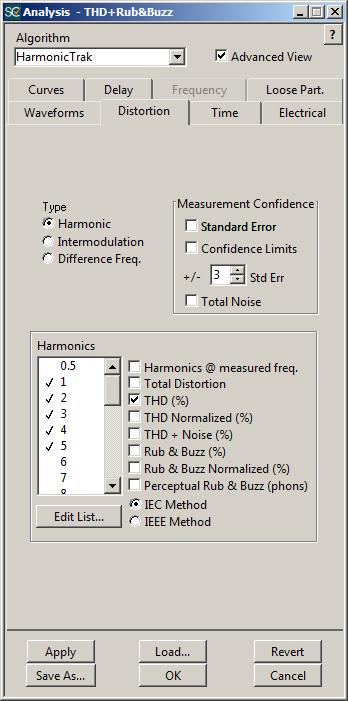Then the THD curve shows the distortion (%) as a result of H2 through H5 compared to the fundamental (H1).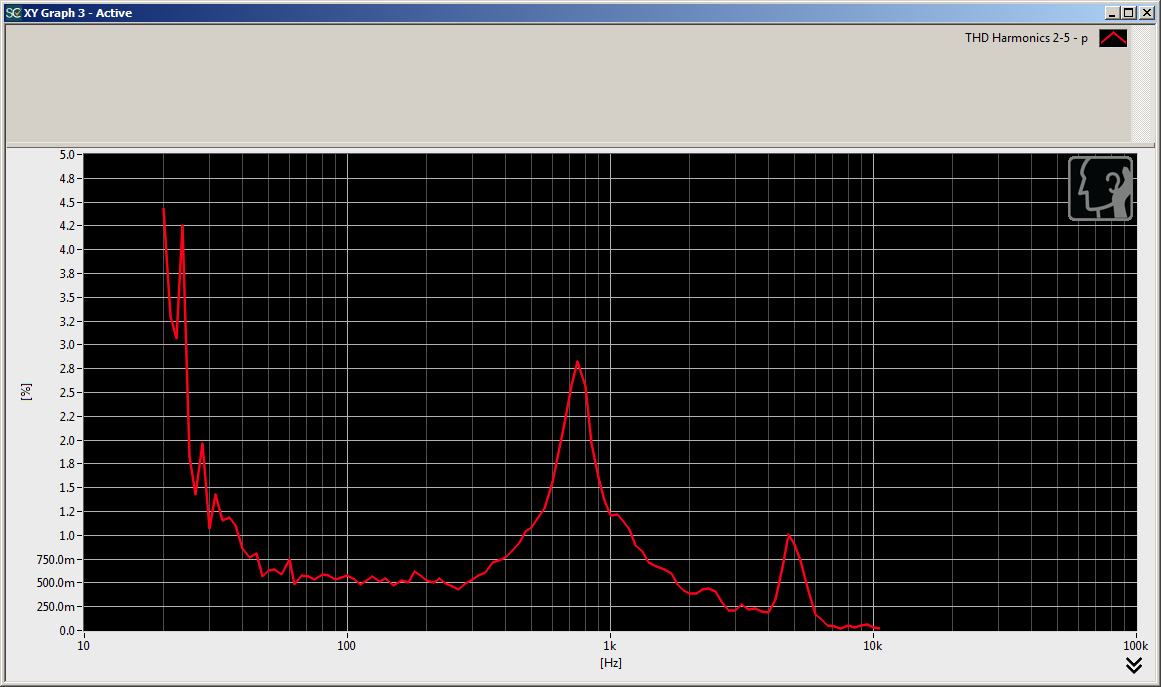Although HarmonicTrak analysis outputs each selected harmonic to the Memory List as a curve using dB units, it is sometimes desirable to look at each harmonic's level expressed as a percentage relative to the fundamental. To view this, simply select the single harmonic of interest in the analysis step.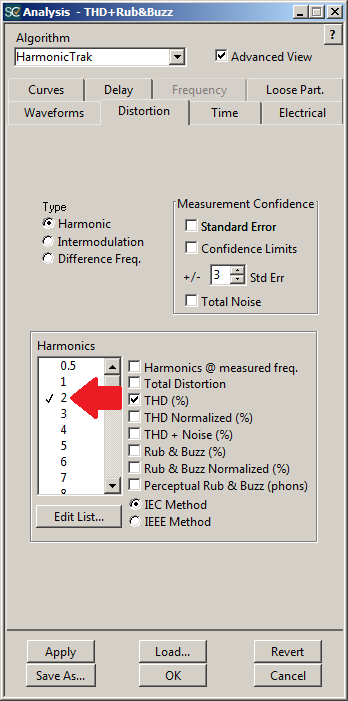This will output a discrete THD curve for that specific harmonic.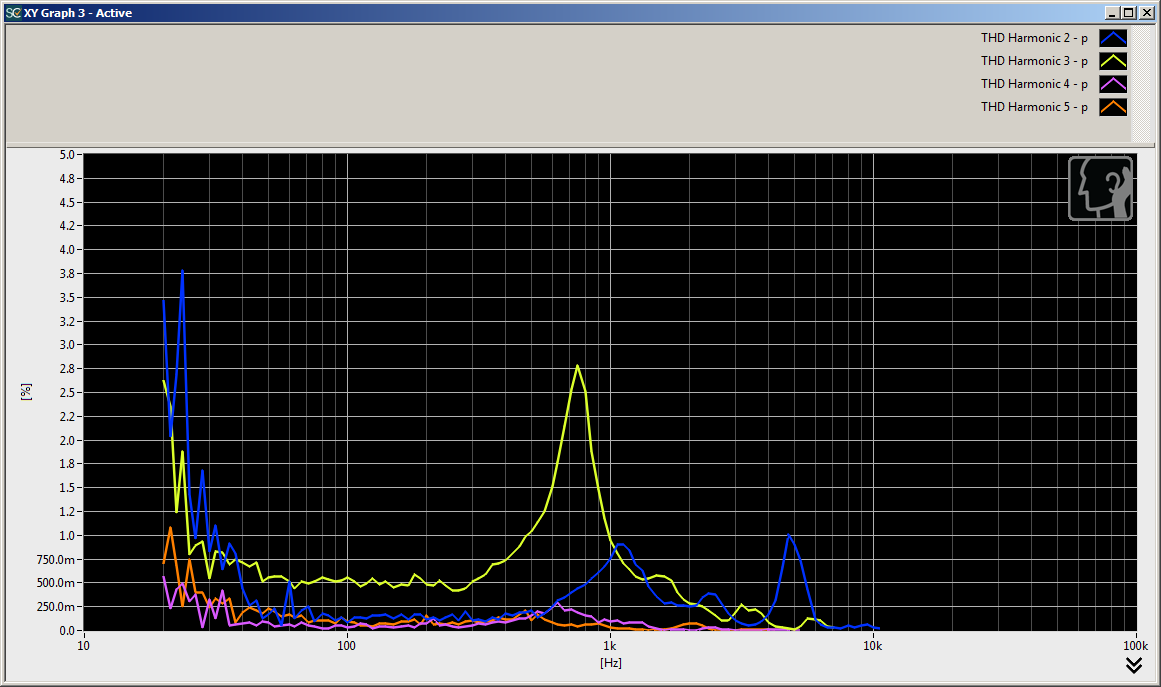Multiple curves can then be compared to the original THD measurement to see which harmonics are producing distortion over certain frequency bands.For example, comparing these curves reveals that the distortion peak at 750Hz is caused by H3 and the peak at 5kHz is caused by H2.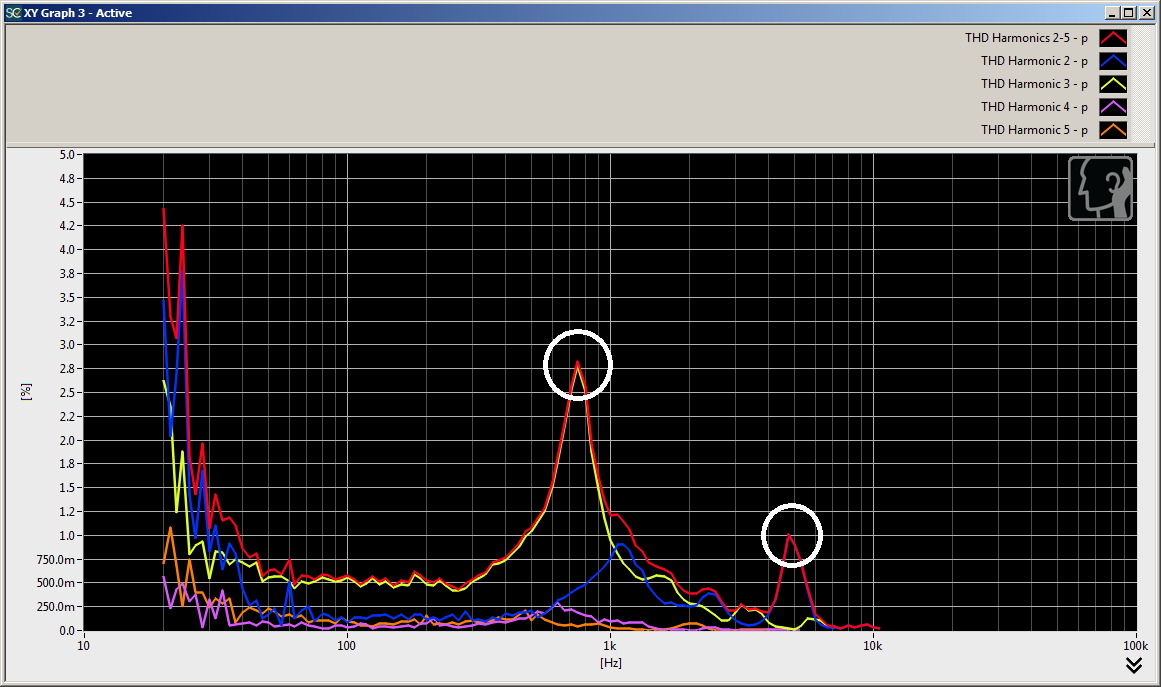0 out of 0 found this helpful
Have more questions? Submit a request

••